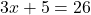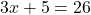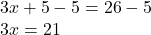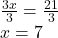## five more than three times a number is 26 what is the number

Question

five more than three times a number is 26 what is the number

in progress 0
2 weeks 2021-09-02T20:10:12+00:00 1 Answers 0 views 0

The required number is 7

Step-by-step explanation:

Let a number = x

We are given the condition: five more than three times a number is 26

We need to find the number.

Writing the condition in mathematical form:

five more than three times a number is 26:Now, solving this equation we can find x, the numberSubtract 5 from both sidesDivide both sides by 3So, we get: x = 7

The required number is 7